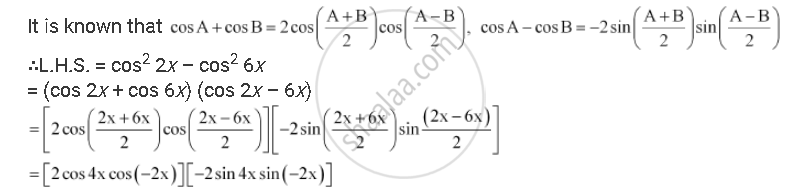CBSE (Arts) Class 11CBSE
Share

# Prove that Cos2 2x – Cos2 6x = Sin 4x Sin 8x - CBSE (Arts) Class 11 - Mathematics

ConceptTrigonometric Functions of Sum and Difference of Two Angles

#### Question

Prove that cos2 2x – cos2 6x = sin 4sin 8x

#### Solution= [2 cos 4x cos 2x] [–2 sin 4(–sin 2x)]

= (2 sin 4x cos 4x) (2 sin 2x cos 2x)

= sin 8x sin 4x

= R.H.S.

Is there an error in this question or solution?

#### APPEARS IN

NCERT Solution for Mathematics Textbook for Class 11 (2018 to Current)
Chapter 3: Trigonometric Functions
Q: 13 | Page no. 73

#### Video TutorialsVIEW ALL 

Solution Prove that Cos2 2x – Cos2 6x = Sin 4x Sin 8x Concept: Trigonometric Functions of Sum and Difference of Two Angles.
S